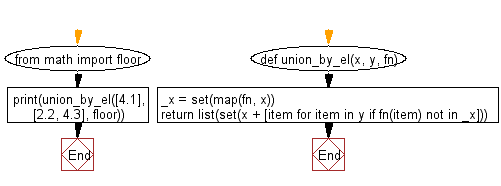﻿ Python: Return every element that exists in any of the two given lists once, after applying function to each element of both - w3resource# Python: Return every element that exists in any of the two given lists once, after applying function to each element of both

## Python List: Exercise - 228 with Solution

Write a Python program to get every element that exists in any of the two given lists once, after applying the provided function to each element of both.

• Create a set by applying fn to each element in x.
• Use a list comprehension in combination with fn on y to only keep values not contained in the previously created set, _x.
• Finally, create a set from the previous result and x and transform it into a list.

Sample Solution:

Python Code:

``````def union_by_el(x, y, fn):
_x = set(map(fn, x))
return list(set(x + [item for item in y if fn(item) not in _x]))
from math import floor
print(union_by_el([4.1], [2.2, 4.3], floor))
```
```

Sample Output:

```[2.2, 4.1]
```

Flowchart:## Visualize Python code execution:

The following tool visualize what the computer is doing step-by-step as it executes the said program:

Python Code Editor:

Have another way to solve this solution? Contribute your code (and comments) through Disqus.

What is the difficulty level of this exercise?

Test your Python skills with w3resource's quiz

﻿

## Python: Tips of the Day

Floor Division:

When we speak of division we normally mean (/) float division operator, this will give a precise result in float format with decimals.

For a rounded integer result there is (//) floor division operator in Python. Floor division will only give integer results that are round numbers.

```print(1000 // 300)
print(1000 / 300)```

Output:

```3
3.3333333333333335```